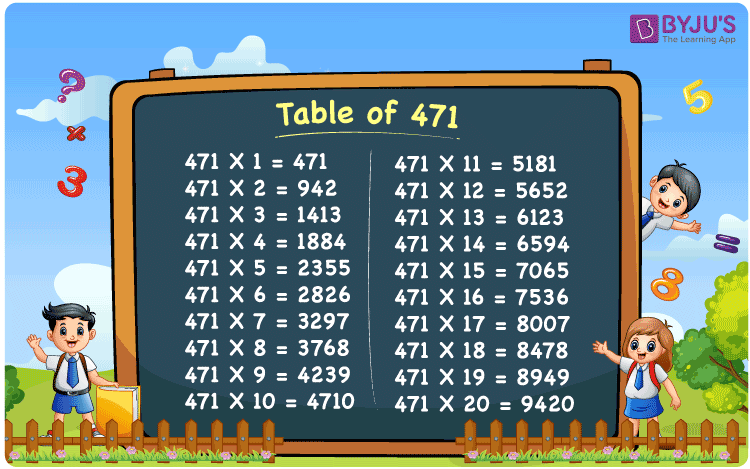Checkout JEE MAINS 2022 Question Paper Analysis : Checkout JEE MAINS 2022 Question Paper Analysis :

# Table of 471

The Table of 471 helps students in their transition from counting their fingers during calculation to mental arithmetic. This is an important skill which should be developed among the students right from their primary education, to build a good understanding of the important concepts of Mathematics. The PDF of the multiplication table of 471 can be downloaded for free by the students by just clicking on the free PDF download option available below.

## Table of 471 Chart## What is the 471 Times Table?

Memorizing the multiplication table of 471 plays an important role in the life of students to speed up their abilities to calculate problems mentally. Maths is a subject which includes a lot of numericals. If a student wastes time in calculating even the multiplication tables manually, 3 hours will not be sufficient to answer the question asked in the exam. To help students in this aspect, the concept of multiplication is discussed in brief in the form of a table below.

 471 × 1 = 471 471 471 × 2 = 942 471 + 471 = 942 471 × 3 = 1413 471 + 471 + 471 = 1413 471 × 4 = 1884 471 + 471 + 471 + 471 = 1884 471 × 5 = 2355 471 + 471 + 471 + 471 + 471 = 2355 471 × 6 = 2826 471 + 471 + 471 + 471 + 471 + 471 = 2826 471 × 7 = 3297 471 + 471 + 471 + 471 + 471 + 471 + 471 = 3297 471 × 8 = 3768 471 + 471 + 471 + 471 + 471 + 471 + 471 + 471 = 3768 471 × 9 = 4239 471 + 471 + 471 + 471 + 471 + 471 + 471 + 471 + 471 = 4239 471 × 10 = 4710 471 + 471 + 471 + 471 + 471 + 471 + 471 + 471 + 471 + 471 = 4710

## Multiplication Table of 471

The Table of 471 is the multiplication of the number 471 with natural numbers up to 20. During an academic year, the main aim of the students is to find the perfect reference material. The highly knowledgeable faculty at BYJU’S prepare the multiplication table of 471 in the form of a chart, table and PDF to meet the requirements of the students.

 471 × 1 = 471 471 × 2 = 942 471 × 3 = 1413 471 × 4 = 1884 471 × 5 = 2355 471 × 6 = 2826 471 × 7 = 3297 471 × 8 = 3768 471 × 9 = 4239 471 × 10 = 4710 471 × 11 = 5181 471 × 12 = 5652 471 × 13 = 6123 471 × 14 = 6594 471 × 15 = 7065 471 × 16 = 7536 471 × 17 = 8007 471 × 18 = 8478 471 × 19 = 8949 471 × 20 = 9420

## Solved Example on the Table of 471

Q.1: 4 dead crows were buried in a day. How many crows will be buried in 471 days?

Solution: Given,

Number of crows buried in a day = 4

In 471 days number of dead crows that will be buried = 471 x 4

= 1884

## Frequently Asked Questions on the Table of 471

### Estimate 471 multiplied by 8.

Using the multiplication table of 471, 471 multiplied by 8 is 471 x 8 = 3768.

### What value has to be multiplied with the number 471 to obtain 7536?

From the table of 471, 471 x 16 = 7536. Therefore, 16 has to be multiplied with the number 471 to obtain 7536.

### Determine the value of 471 times 13 plus 6 minus 17.

In the table of 471, 471 times 13 plus 6 minus 17 can be written as 471 x (13 + 6 – 17) = 471 x 2 = 942